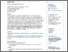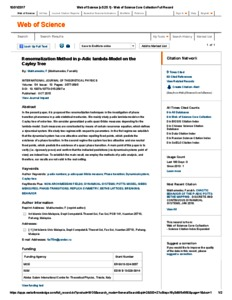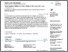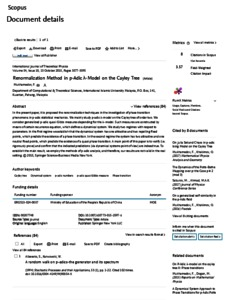# Renormalization method in p-adic λ-model on the Cayley tree

Mukhamedov, Farrukh (2015) Renormalization method in p-adic λ-model on the Cayley tree. International Journal of Theoretical Physics, 54 (10). pp. 3577-3595. ISSN 0020-7748PDF - Published Version
Restricted to Registered users onlyPreview
PDFPreview
PDF

## Abstract

In this present paper, it is proposed the renormalization techniques in the investigation of phase transition phenomena in p-adic statistical mechanics. We mainly study p-adic $\l$-model on the Cayley tree of order two. We consider generalized p-adic quasi Gibbs measures depending on parameter $\r\in\bq_p$, for the $\l$-model. Such measures are constructed by means of certain recurrence equations. These equations define a dynamical system. We study two regimes with respect to parameters. In the first regime we establish that the dynamical system has one attractive and two repelling fixed points, which predicts the existence of a phase transition. In the second regime the system has two attractive and one neutral fixed points, which predicts the existence of a quasi phase transition. A main point of this paper is to verify (i.e. rigorously prove) and confirm that the indicated predictions (via dynamical systems point of view) are indeed true. To establish the main result, we employ the methods of p-adic analysis, and therefore, our results are not valid in the real setting.

Item Type: Article (Journal) 5537/44980 p-adic numbers · p-adic quasi Gibbs measure · Phase transition · Dynamical system · Cayley tree Q Science > QA Mathematics Kulliyyah of Science > Department of Computational and Theoretical Sciences Dr. Farrukh Mukhamedov 08 Oct 2015 09:36 31 Oct 2017 09:39 http://irep.iium.edu.my/id/eprint/44980View Item